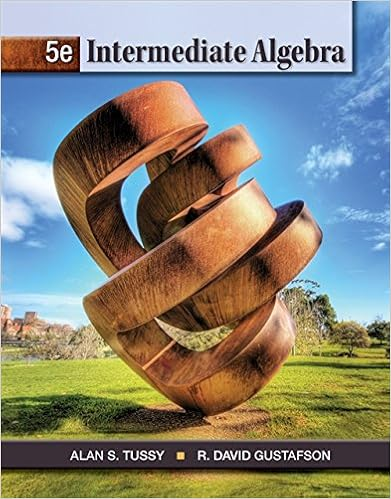# MAT 353 Assignment3 - 3.3 a >...

• Homework Help
• 5

This preview shows page 1 - 3 out of 5 pages.

##### We have textbook solutions for you!
The document you are viewing contains questions related to this textbook.The document you are viewing contains questions related to this textbook.
Chapter 11 / Exercise 9
Intermediate Algebra
Tussy/GustafsonExpert Verified
3.3a.> data<-read.table("%20%201%20Data%20Sets/CH01PR19.txt")> View(data)> boxplot(data\$V2)The data appears fairly normal, and there are no real outliers.b.> dotchart(data\$V2)The dot plot has random scatter with fairly constant variance.c. > lmfit<-lm(data)> summary(lmfit)Call:lm(formula = data)Residuals:Min 1Q Median 3Q Max -2.74004 -0.33827 0.04062 0.44064 1.22737 Coefficients:Estimate Std. Error t value Pr(>|t|) (Intercept) 2.11405 0.32089 6.588 1.3e-09 ***ACT 0.03883 0.01277 3.040 0.00292 ** ---Signif. codes: 0 ‘***’ 0.001 ‘**’ 0.01 ‘*’ 0.05 ‘.’ 0.1 ‘ ’ 1Residual standard error: 0.6231 on 118 degrees of freedomMultiple R-squared: 0.07262,Adjusted R-squared: 0.06476
##### We have textbook solutions for you!
The document you are viewing contains questions related to this textbook.The document you are viewing contains questions related to this textbook.
Chapter 11 / Exercise 9
Intermediate Algebra
Tussy/GustafsonExpert Verified
F-statistic: 9.24 on 1 and 118 DF, p-value: 0.002917> plot(lmfit)Hit <Return> to see next plot: Hit <Return> to see next plot: Hit <Return> to see next plot: Hit <Return> to see next plot: The plot appears to be the ideal case for regression. There is no apparent pattern in the residuals and constant variance.d.> qqnorm(resid(lmfit))> qqline(resid(lmfit))> GPA.quantiles = qqnorm(resid(lmfit))> GPA.quantiles.lm = lm(y~x, data=GPA.quantiles)
•••